Next: 3.3 Hash Tables Up: 3. Dictionaries Previous: 3.1 Sets

# 3.2 Dictionaries

• A dictionary is a dynamic set ADT with the operations:
1.
Makenull (D)
2.
Insert (x, D)
3.
Delete (x, D)
4.
Search (x, D)

• Useful in implementing symbol tables, text retrieval systems, database systems, page mapping tables, etc.

• Implementation:
1.
Fixed Length arrays
2.
Linked lists : sorted, unsorted, skip-lists
3.
Hash Tables : open, closed
4.
Trees

• Binary Search Trees (BSTs)
• Balanced BSTs
• AVL Trees
• Red-Black Trees
• Splay Trees
• Multiway Search Trees
• 2-3 Trees
• B Trees
• Tries
• Let n be the number of elements is a dictionary D. The following is a summary of the performance of some basic implementation methods:

 Worst case complexity of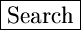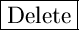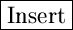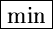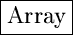O(n) O(n) O(n) O(n)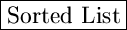O(n) O(n) O(n) O(1)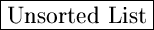O(n) O(n) O(n) O(n)

Among these, the sorted list has the best average case performance.

• In this chapter, we discuss two data structures for dictionaries, namely Hash Tables and Skip Lists.Next: 3.3 Hash Tables Up: 3. Dictionaries Previous: 3.1 Sets
eEL,CSA_Dept,IISc,Bangalore